# How to Copy Rows Automatically in Excel to Another Sheet (4 Methods)

Get FREE Advanced Excel Exercises with Solutions!

In this tutorial, we will demonstrate the methods to automatically copy rows in Excel to another sheet. Microsoft Excel does not provide any feature to copy rows automatically to another sheet. So, I have to go through multiple steps to copy rows from one sheet to another.

## How to Copy Rows Automatically in Excel to Another Sheet: 4 Methods

To copy rows automatically in Excel to another sheet we can apply multiple methods. In this tutorial, we will explain 4 methods to copy rows to another sheet.

### 1. Automatically Copy Rows in Excel to Another Sheet Using Filters

First and foremost, we will use the filter option to copy rows automatically. In the following dataset, we will copy all rows from the data range (B4:D10) except the rows that have sales data for “New York” city.Let’s go through the steps to perform this action.

STEPS:

• Firstly, select the data range (B4:D10).
• Then got to the Data From the drop-down of the “Sort & Filter” option select the option “Filter”.
• In short: Select data range (B4:D10) > Data tab > Sort & Filter > Filter• The above action makes the filter icon visible in the header of the data range.• Secondly, click on the drop-down icon in cell D4.
• Check only the option New York and uncheck the rest of all.
• Press OK.• The above command returns the filtered value of the selected data range. We can see only the rows having the value of city New York.• Thirdly, select the data range (B4:D10) and press Alt +; to select all the visible cells in the filtered data range.
• Then do a right-click and from the popup menu select the option Copy or we can use the shortcut CTRL+C to copy the selected data range.• Fourthly, go to another sheet named “Copy-Filter” and paste the copied data.• After that, go back to the previous sheet, select cells (B5:D10), and delete them.• So, we can see there is no data available in our worksheet now.• Lastly, go to the Data tab and disable the filter option. So, we get the sales data of the city New York deleted from the data range.NOTE:

This method is partially automatic. Because at one stage we have to copy and paste data manually. So, this method is not so feasible in real-time.

### 2. Combine IFERROR & VLOOKUP Functions to Copy Rows Automatically to Another Sheet in Excel

We can easily copy rows automatically in Excel by using the combination of the IFERROR with VLOOKUP functions. The IFERROR function is applied to intercept errors and returns a defined value if the formula in this function occurs error. VLOOKUP is an abbreviation for ‘Vertical Lookup’. It allows Excel to look for a specific value in a column so that it can return a value from another column in the same row. To illustrate this method we will continue with the same dataset that we used in the previous example.

###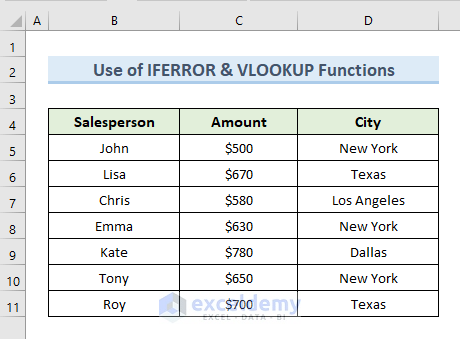So, let’s take a look at the steps to perform this action.

STEPS:

• First, go to the sheet IFERROR &VLOOKUP(2) where we want to paste our data.
• Next, select cell B4 and insert the following formula in that cell:
`=IFERROR(VLOOKUP('IFERROR &VLOOKUP'!\$B\$4:\$B\$1000,'IFERROR &VLOOKUP'!\$B\$4:\$D\$1000,1, FALSE), "")`

###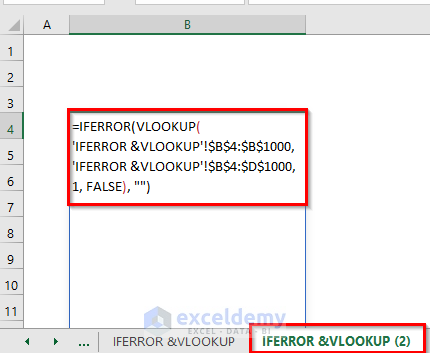• Now, press ENTER. The above command returns the values of column B from the sheet “IFERROR & VLOOKUP” in sheet “IFERROR &VLOOKUP (2)”.

###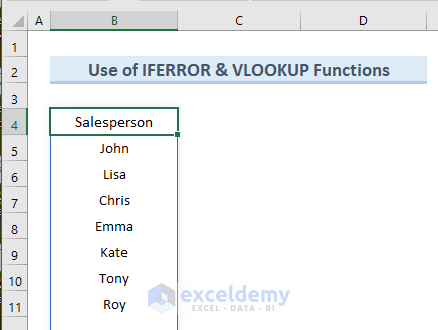• Similarly, insert the following formulas in cells C4 and D4 of the worksheet IFERROR &VLOOKUP (2)”.

In cell C4 insert the formula is:

`=IFERROR(VLOOKUP('IFERROR &VLOOKUP'!\$B\$4:\$B\$1000,'IFERROR &VLOOKUP'!\$B\$4:\$D\$1000,2, FALSE), "")`

In cell D4 insert the formula is:

`=IFERROR(VLOOKUP('IFERROR &VLOOKUP'!\$B\$4:\$B\$1000,'IFERROR &VLOOKUP'!\$B\$4:\$D\$1000,3, FALSE), "")`
• So, the above formulas will give you a dataset like the following image.

###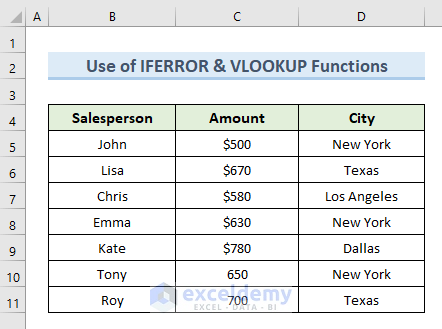• After that, go back to the worksheet IFERROR &VLOOKUP. Add a new row in the data range like the highlighted one in the following image.

###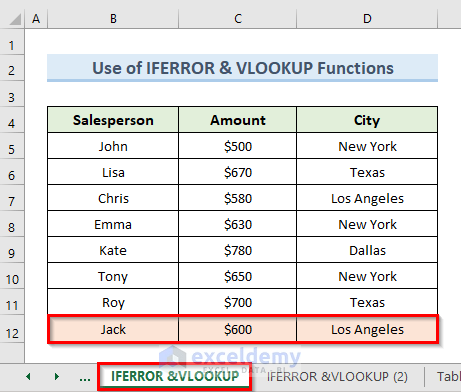• Finally, open the worksheet IFERROR &VLOOKUP(2) Here we can see that the newly added row in the worksheet IFERROR &VLOOKUP is duplicated into the worksheet IFERROR &VLOOKUP(2) as seen above.

###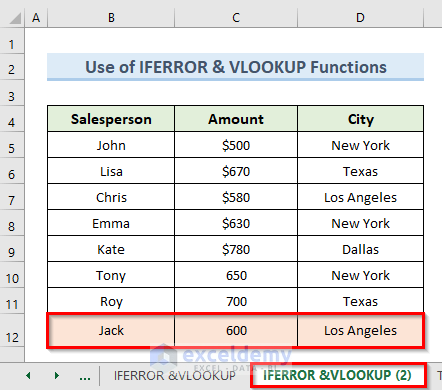🔎 How Does the Formula Work?

• VLOOKUP(‘IFERROR &VLOOKUP’!\$B\$4:\$B\$1000,’IFERROR &VLOOKUP’!\$B\$4:\$D\$1000,1, FALSE), “”): This part retrieve the values from the first column of the worksheet IFERROR &VLOOKUP.
• IFERROR(VLOOKUP(‘IFERROR &VLOOKUP’!\$B\$4:\$B\$1000,’IFERROR &VLOOKUP’!\$B\$4:\$D\$1000,1, FALSE), “”): Returns blank if any VLOOKUP value in the range (\$B\$4:\$D\$1000) gives an error.

### 3. Insert Table Query Window to Copy Rows Automatically in Excel to Another Sheet

In this example, we will use the “Table Query Window” to copy rows automatically to another sheet in Excel. To apply this method we will continue with our previous dataset. But for this method, we will use the table format of the data range like the following image.

###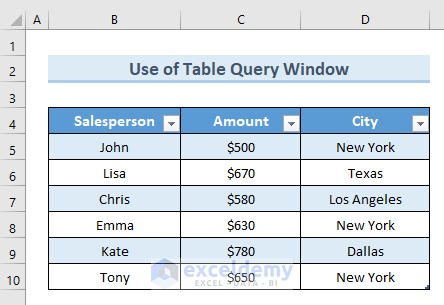So, let’s go through the steps associated with the method.

STEPS:

• In the beginning, select the entire table range (B4:D10).
• Then go to the Table Design tab and select the option Properties. We will rename the table as “New_Table”.

###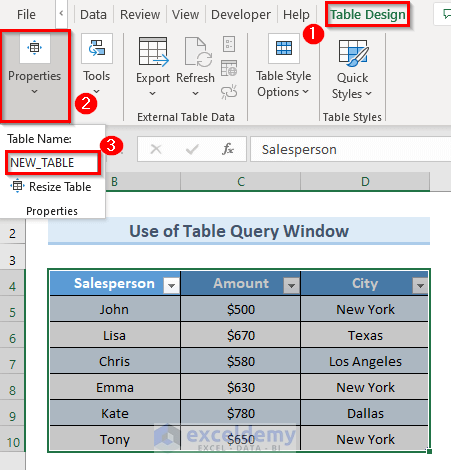• Next, Select the option From Table/Range from the ribbon of the Data tab.

###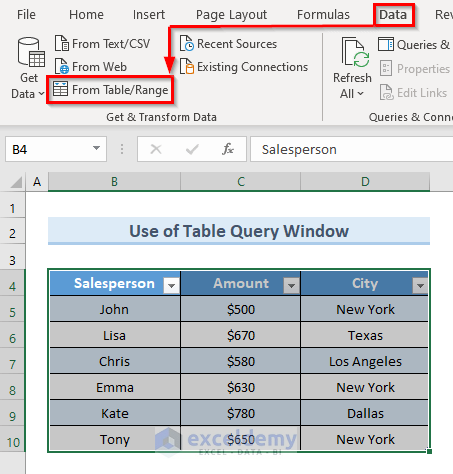• Now a new window will appear for query settings.
• Go to the Add Column tab in that window. From the drop-down of the Index Column select the option From 1.

###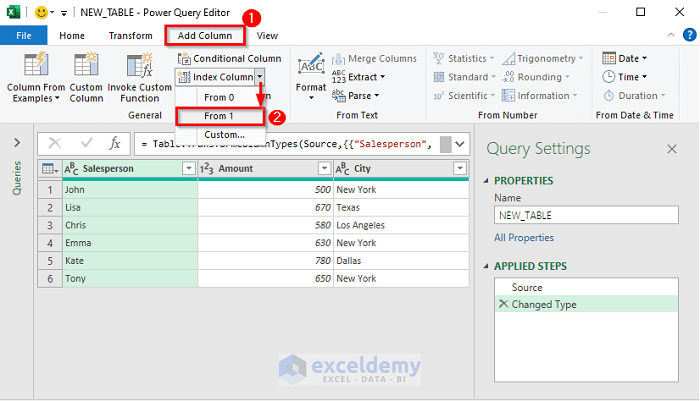• So, the above action adds a new Index column with our existing data range.

###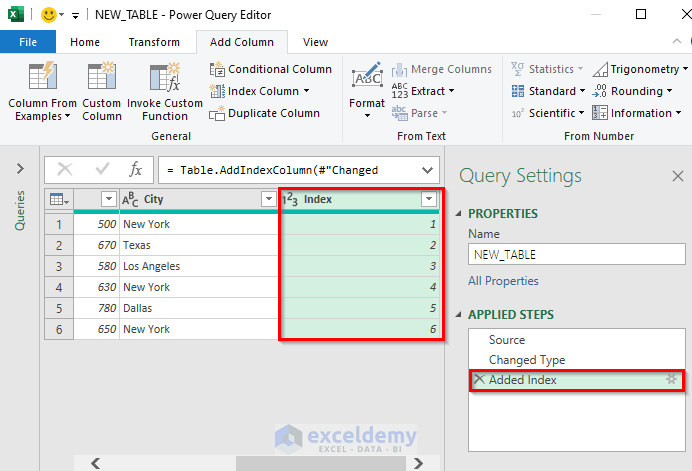• Next, go to the File Click on the option “Close & Load To”.

###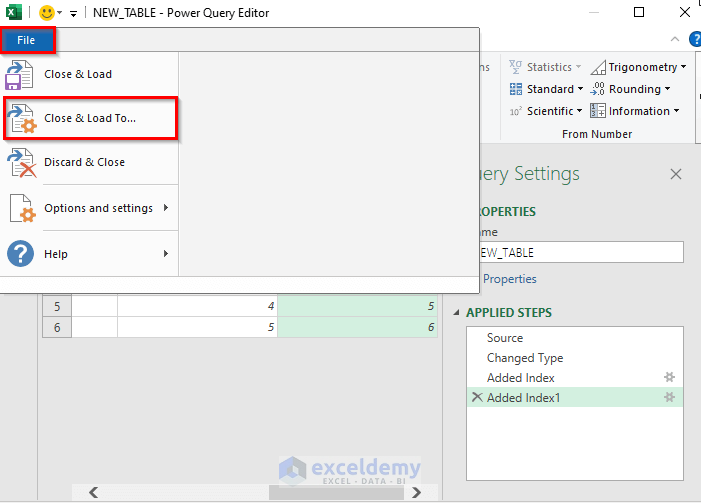• One more dialogue box will appear. In that box check the option “New worksheet” to put the data in a new worksheet. Also, check the option “Add this data to the Data Model”.
• Then, press OK.

###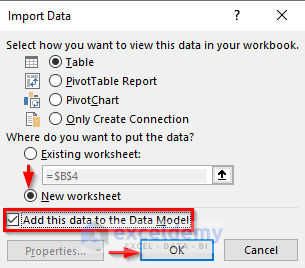• The above commands return a new table in a new worksheet. We can see that the new worksheet’s name is the same as the table’s name.

###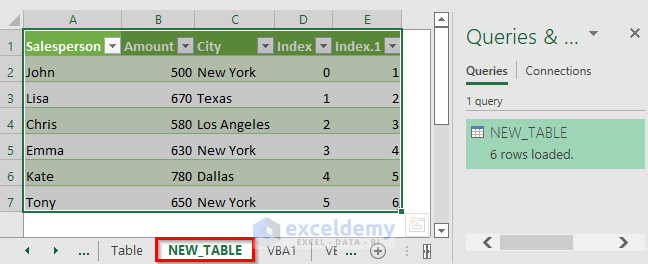• Then, go back to the worksheet “Table”. Add a new row in the data range like the highlighted one in the following image.

###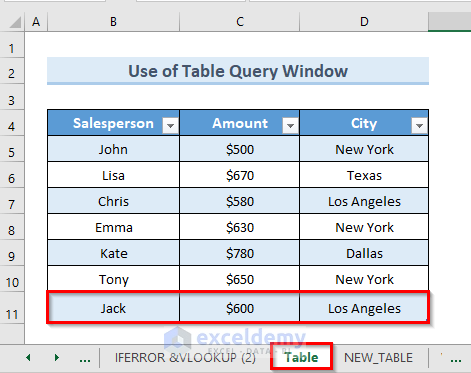• After that, again go to the worksheet “NEW_TABLE”. Right-click on the name of the worksheet in the “Queries & Settings” section and click on “Refresh”.

###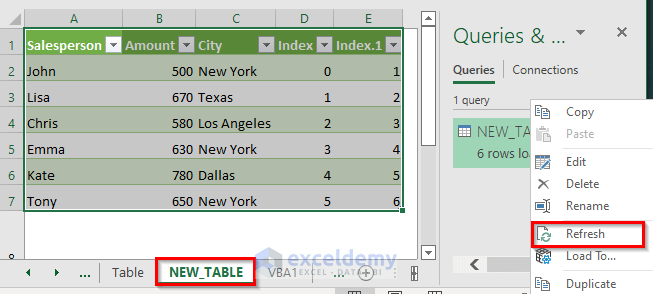• Lastly, we can see that if we add a new row in the table of the worksheet named “Table” it is automatically duplicated into the new worksheet that we get from “Power Query Editor”.

### 4. VBA Code to Copy Rows Automatically in Excel to Another Sheet

Using VBA code to copy rows automatically in Excel to another sheet is the most effective way. We will demonstrate 3 ways to apply VBA code to copy rows from a dataset.

#### 4.1 Keep Source Data and Copy Rows Automatically to Another Sheet in Excel

We’ll use VBA code to copy rows to another sheet the first way. This method not only copies the rows to another sheet but also keeps the source data unchanged. In this example, we will copy the rows from the dataset given below.

####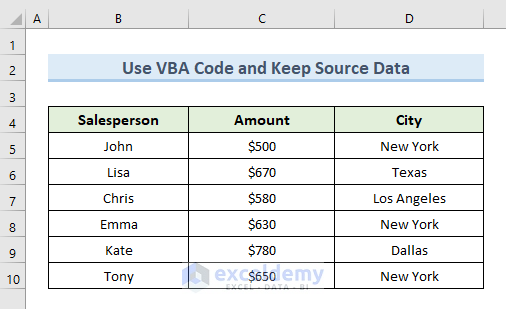So, let’s go through the steps to perform this action.

STEPS:

• Firstly, right-click on the sheet name from which we want to copy rows. Select the option “View Code”.

####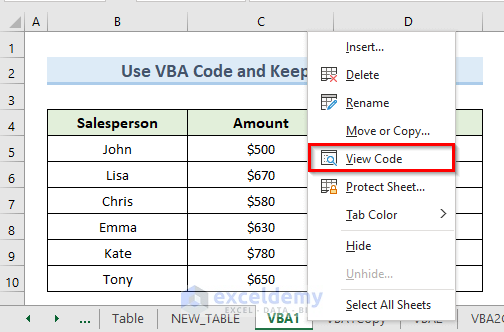• A new blank VBA module will appear.
• Secondly, insert the following code in the blank window:
``````Sub copy_row_1()
Dim r1 As Range
Dim r2 As Range
Set r1 = ThisWorkbook.Sheets("VBA1").UsedRange
r2.Value = r1.Value
End Sub``````
• Then click on Run.
• This code will copy the rows from sheet “VBA1” to “VBA1Copy”. We can see the sheet names are highlighted in the below image.

####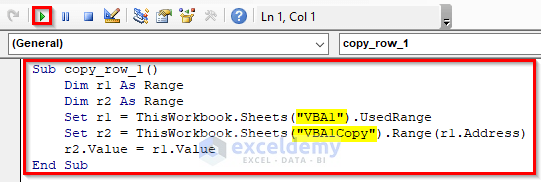• Lastly, go to the sheet “VBA1Copy”. We can see that in sheet “VBA1Copy” all of the rows from sheet “VBA1” are copied. If we go back to sheet “VBA1” we will see the source data is unchanged.

#### 4.2 Automatically Copy Rows to Another Sheet and Remove from Source Data in Excel

In this method, the original value, however, will be removed after copying rows from a worksheet. We will copy only the rows in the dataset below that have the value “New York” in the column City.

####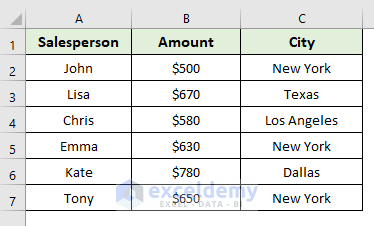Let’s look at the steps that go along with this method.

STEPS:

• First, select the data range (A1:C7).
• Next, right-click on the worksheet name “VBA2”. Click on the “View Code”

####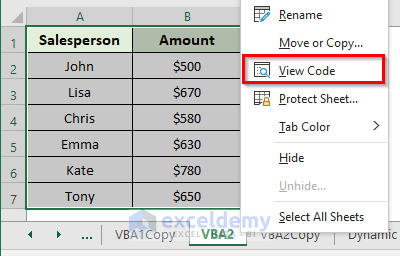• Now, we can see a new blank VBA.
• Then, insert the following code in the blank window:
``````Sub copy_rows()
For Each cell In Selection.Columns(3).Cells
If cell.Value = "New York" Then
cell.EntireRow.Copy Worksheets("VBA2Copy").Range("A" & Rows.Count).End(3)(2)
cell.EntireRow.Delete
End If
Next
End Sub``````
• Click on Run.
• This code will copy the rows from sheet “VBA2” to “VBA2Copy”. We can see the sheet names are highlighted in the below image.

####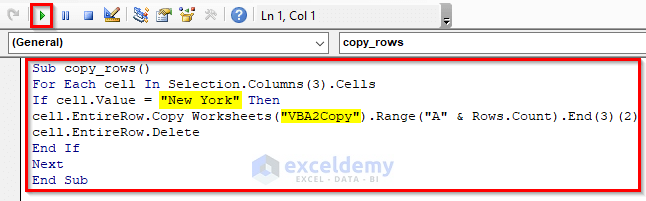• After that, we can see that this action deletes the row from the sheet “VBA2” that has the value “New York” in the column City.

####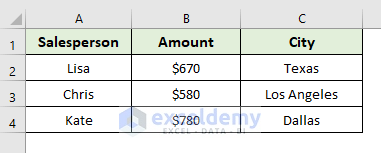• Finally, if we go to sheet “VBA2Copy” we can see that all the rows with the value “New York” in column City are copied in that sheet.

#### 4.3 Copy Rows in Excel to Another Sheet Dynamically

In this method, we will apply a VBA code in our previous dataset to copy rows. This time the code will group the values of column City by their name. Then it will copy the values with the same name to an individual worksheet.So, let’s have a look at the steps involved in this method.

STEPS:

• Firstly, select the sheet Dynamic. Right-click on the sheet and select the option “View Code”.• Now, we can see a new blank VBA.
• Secondly, insert the following code in the blank window:
``````Sub Copy_Rows_3()
Dim r1 As Range, Row_Last As Long, sht As Worksheet
Dim Row_Last1 As Long
Dim src As Worksheet
'Change this to the sheet with the data on
Set src = Sheets("Dynamic")
Row_Last = src.Cells(Cells.Rows.Count, "B").End(xlUp).Row
For Each r1 In src.Range("B5:B10" & Row_Last)
On Error Resume Next
Set sht = Sheets(CStr(r1.Value))
On Error GoTo 0
If sht Is Nothing Then
'Sheets(CStr(r.Value)).Cells(1, 1) = "Total"
Row_Last1 = Sheets(CStr(r1.Value)).Cells(Cells.Rows.Count, "B").End(xlUp).Row
src.Rows(r1.Row).Copy Sheets(CStr(r1.Value)).Cells(Row_Last1 + 1, 1)
Sheets(CStr(r1.Value)).Cells(1, 2) = WorksheetFunction.Sum(Sheets(CStr(r1.Value)).Columns(3))
Set sht = Nothing
Else
'Sheets(CStr(r.Value)).Cells(1, 1) = "Total"
Row_Last1 = Sheets(CStr(r1.Value)).Cells(Cells.Rows.Count, "B").End(xlUp).Row
src.Rows(r1.Row).Copy Sheets(CStr(r1.Value)).Cells(Row_Last1 + 1, 1)
Sheets(CStr(r1.Value)).Cells(1, 2) = WorksheetFunction.Sum(Sheets(CStr(r1.Value)).Columns(3))
Set sht = Nothing
End If
Next r1
End Sub``````
• Click on Run.• This code will copy the rows from sheet “Dynamic” to individual sheets. We can see that the individual sheet is named after the values of column City.• If we click on sheet “New York” we can see only the rows that have the value “New York” in this sheet.• Then, click on sheet “Texas” we can see only the rows that have the value “Texas” in this sheet.• Finally, click on sheet “Los Angeles”, we can see only the rows that have the value “Los Angeles” in this sheet.## Conclusion

We’ve covered how to automatically copy rows in Excel to another sheet in this article. Hopefully, the previous examples have clarified the process to copy rows automatically. Use the practice worksheet that comes with this article to put your skills to the test. If you have any questions, please leave a comment in the box below. Our team will try to respond as soon as possible.

## What is ExcelDemy?

ExcelDemy Learn Excel & Excel Solutions Center provides free Excel tutorials, free support , and premium Excel consultancy services for Excel and business users. Feel free to contact us with your Excel projects.Mukesh Dipto

Hi there! I am Mukesh Dipto. I graduated with a bachelor's degree in engineering. Currently, I am working as a technical content writer in ExcelDemy. You will find all my articles on Microsoft Excel on this site. Outside of the workplace, my hobbies and interests include sports and meeting new people. I also enjoy sports. My favorite sports are football (to watch and play) and Badminton (play).

1. ReplyIs there a way to do this for multiple values to go to a different tab?

Example: I have a list of employees with different types of employment; Federal, Casual Hire, Contract, Forest Service, etc.

I have my main sheet (Master), I have column E that classifies which type of employee they are, I want that row to copy to a new tab depending on which group they are in.

In other words, I would like a tab for Federal with all the federal from column E to copy into it, a tab for casual hire with all the casual hire from column E, etc.

2. ReplyZehad Rian Jim Aug 17, 2022 at 12:39 PM

Hello DESTINY,
First, thanks for your curious question. It was amusing to solve the problem. Let me guide you to fulfill your query.

Step 1. Assume you have a Dataset where you have the Names of the employees in one column and the types of the employees in another.Step 2. Then insert the following code in the VBA window.

Sub Copy_Rows_3()
Dim r1 As Range, Row_Last As Long, sht As Worksheet
Dim Row_Last1 As Long
Dim src As Worksheet
‘Change this to the sheet with the data on
Set src = Sheets(“Dynamic”)
Row_Last = src.Cells(Cells.Rows.Count, “C”).End(xlUp).Row
For Each r1 In src.Range(“C5:C13” & Row_Last)
On Error Resume Next
Set sht = Sheets(CStr(r1.Value))
On Error GoTo 0
If sht Is Nothing Then
‘Sheets(CStr(r.Value)).Cells(1, 1) = “Total”
Row_Last1 = Sheets(CStr(r1.Value)).Cells(Cells.Rows.Count, “B”).End(xlUp).Row
src.Rows(r1.Row).Copy Sheets(CStr(r1.Value)).Cells(Row_Last1 + 1, 1)
Sheets(CStr(r1.Value)).Cells(1, 2) = WorksheetFunction.Sum(Sheets(CStr(r1.Value)).Columns(3))
Set sht = Nothing
Else
‘Sheets(CStr(r.Value)).Cells(1, 1) = “Total”
Row_Last1 = Sheets(CStr(r1.Value)).Cells(Cells.Rows.Count, “B”).End(xlUp).Row
src.Rows(r1.Row).Copy Sheets(CStr(r1.Value)).Cells(Row_Last1 + 1, 1)
Sheets(CStr(r1.Value)).Cells(1, 2) = WorksheetFunction.Sum(Sheets(CStr(r1.Value)).Columns(3))
Set sht = Nothing
End If
Next r1
End Sub

N.B. if you are following our article, use the VBA code under the method “Copy Rows in Excel to Another Sheet Dynamically” and change the marked portions.Step 3. After pressing Run, you will get the result in individual desired cells.Thanks

3. ReplyGood morning,

I am trying to update an existing excel file to make it a bit more efficient. At this point I am having to copy, paste and assort data from an Access file. I am looking to take the data from the Access file, place a copy of it on the source sheet, and then have excel automatically put a copy of each row on their appropriate sheets based on the plant listed on column “L”.

I have downloaded the example excel file and attempted the example/instructions you give in 4.2. However, when I try this on either my excel or yours I get the errors code ‘1004’

If you have any idea what is causing this and it’s solution, I would sincerely appreciate your help. Thanks

4. ReplyZEHAD RIAN JIM Aug 22, 2022 at 11:02 AM

Hello MICHAEL,
Thanks for the amazing question. Let me guide you in solving this problem.

If you want to solve this problem at first you have to know the possible reason behind this problem.
The first reason can be MACRO NAME ERROR.
This problem occurs if VBA code is used but the file has not been saved as ‘Macro-Enabled Workbook’.
The second reason can be FILE CONFLICT.
This problem occurs when the file has many codes and somehow they get conflicted with each other.
Another reason can be “TOO MUCH LEGEND ENTRIES”
If the excel chart has more legend entries than the space available then this problem occurs.
The last possible reason can be “EXCEL FILE CORRUPTION”
In this case, the whole file got corrupted or damaged, or infected.

Though many reasons can cause this error 1004 problem luckily we can fix it with the help of some easy methods.

Solution 1. Deleting GWXL97.XLA Files
Step 1. Go to C:\Program Files\MS Office\Office\XLSTART.
Step 2. Find GWXL97.XLA file and delete it.
Step 3. After deleting the file, reopen the excel file and check if the problem is solved or not.

Solution 2. Check Trust Access
Step 1. Open the excel file.
Step 2. Click on the Files option and press Option.
Step 3. Go to the ‘Trust Center Option’ and enter into the ‘Trust Center Settings’ option.
Step 4. After that go to the ‘Developer Macro Settings’ and click the tick option and check if the problem is solved or not.

Solution 3. Create Another ‘Macro-Enabled Workbook Template’
Step 1. Open a new workbook and entry the data as before.
Step 2. Go to File>Save As options.
Step 3. In the File name section, select the Excel Macro-Enabled Workbook option.
Step 4. Save the file with the desired name.

These solutions should solve your problem. If you face any further problems, please let us know so that we can help you. Thanks.

5. ReplyHi Rian,

I am able to run the code but it is only fetching first row and created sheet but not populating the second row. its giving run time error 1004

• ReplyHey Bharat, thanks for reaching out. Could you specify the code that face problem using it?

6. ReplyI have more complicated situation. I’m not sure it’s even possible to do but maybe you can help me. I have 1 Sheet full of data and 2 sheet full of data. I want to merge these sheets, so transfer data from one sheet to another. But the transfer should happened into the specific rows. For example:
Data from Sheet 1 (A10072:AT10801) has to go to Sheet 2 (A10772:AT10801) and data from Sheet 1 (A10802:AT10831) has to go to Sheet 2 (A10802:AT10831). Simple formula and clicking and dragging would not work as its too much time wasted. Maybe you have a solution for me

• ReplyOsman Goni Ridwan Sep 12, 2022 at 9:29 AM

Hello JUSTINA!
I have an easy solution to your problem. To copy data of range Sheet1 (A10072:AT10801) to range Sheet 2 (A10772:AT10801), you can use this VBA Code.

``````Sub copy_to_another_worksheet()
Worksheets("Sheet1").Range("A10072:AT10801").Copy _
Worksheets("Sheet2").Range("A10772:AT10801")
End Sub``````

And to copy data of range Sheet 1 (A10802:AT10831) to the range Sheet 2 (A10802:AT10831), use the following VBA code-

``````Sub copy_to_another_worksheet()
Worksheets("Sheet1").Range("A10802:AT10831").Copy _
Worksheets("Sheet2").Range("A10802:AT10831")
End Sub``````

I hope, your problem will be solved in this way. You can share more problems in an email at [email protected]

7. ReplyHello!

I was successful in using your instructions here- 4.3 Copy Rows in Excel to Another Sheet Dynamically to have my data filter to In-Progress or Complete tabs. Two problems and/or questions you may be able to assist with.

1. Is there anyway to make this process not duplicate data which has already copied over. For example. If I add a row of data to the dynamic tab and run the process it copies overall all the data not just the new data added.

2. I was trying to also add separate code or logic to the In Progress tab that if Column M changes to complete it would delete it from this tab and move it to the Complete tab. I can’t seem to get this to work even when using your notes here 4.2 Automatically Copy Rows to Another Sheet and Remove from Source Data in Excel. I am not getting an error message, simply nothing happens.

Any help to these 2 issues would be greatly appreciated!

8. ReplyZehad Rian Jim Sep 23, 2022 at 1:01 AM

Hello CALEY FORBES,
Thanks for the amazing question. Let me guide you in solving this problem.

First, I want to solve your first query. I think to solve your problem it is better to use our first method ‘Automatically Copy Rows in Excel to Another Sheet Using Filters’ method than using VBA code. The reason behind this is I think the first method will do your desired work without hesitation.

For the second query, you have to insert the following formula in the VBA windows.

Sub Cut_Range_To_Clipboard()
Range(“B4:B10”).Cut ‘This will cut the source range and copy the Range “B4:B10” data into Clipboard
‘Now you can select any range and paste there
Range(“J2”).Select
ActiveSheet.Paste
End Sub

Note: in the Range section you can change the desired option to paste accordingly.

I hope, your problem will be solved in this way. You can share more problems in an email at [email protected]
Happy Excelling!!!

9. ReplyHello,

I have tried to use the code for Copying Rows in Excel to Another Sheet Dynamically and it is working well except that it creates a blank sheet and getting an error code 400. Are there other variables/ranges that I need to adjust?

Regards,

10. ReplyHello again,

Just to add to my question, I am getting an error on the line below.

Regards,

• ReplyNaimul Hasan Arif Oct 11, 2022 at 7:32 PM

Actually, there is just a little mistake in the code. Instead of writing B5:B, it’s been written B5:B10. This correction has worked perfectly for me.You can submit more problems to us at [email protected]. Regards!

11. ReplyIs there a way to make this code only copy values and not formulas?

For Each cell In Selection.Columns(3).Cells
If cell.Value = “New York” Then
cell.EntireRow.Copy Worksheets(“VBA2Copy”).Range(“A” & Rows.Count).End(3)(2)
End If
Next
End Sub

• ReplyHello, Rick!
Thanks for sharing your problem with us!
While copying, you have to copy the whole thing (values + formulas). You can have the values (without any formula) only while pasting the copied portion into another place.
While pasting, instead of using ‘.Paste‘ to replicate a formula result as a value rather than the formula itself, use ‘.PasteSpecial‘.

``````For Each cell In Selection.Columns(3).Cells
If cell.Value = “New York” Then
cell.EntireRow.Copy
Worksheets(“VBA2Copy”).Range(“A” & Rows.Count).End(3)(2).PasteSpecial xlPasteValues
End If
Next``````

Good Luck!

Regards,
Sabrina Ayon
Author, ExcelDemy
.Advanced Excel Exercises with Solutions PDF